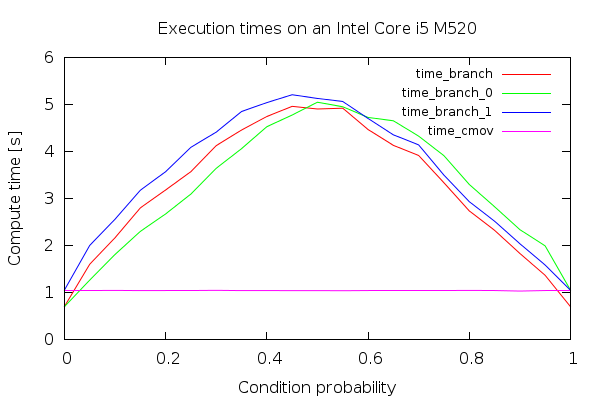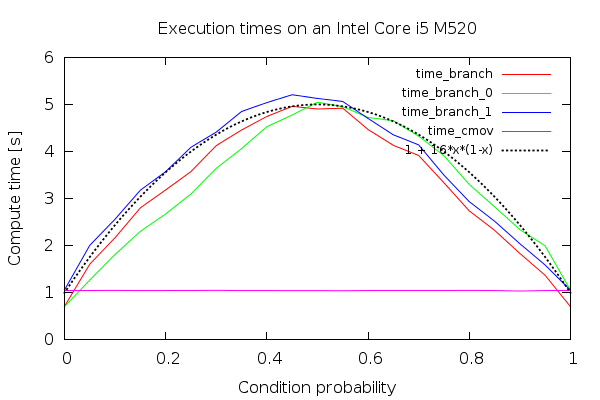# Just how good is the x86 cmov instruction?

Suppose you want to write the following code inside a performance critical loop:

``````if(cond) {
foo = bar;
}
``````

where `foo` is a local variable (of some simple type, like `int`), and `bar` is either a local variable, or a simple memory location (such as `A[i]`). Such a code is likely to be found for example in a Kadane's algorithm implementation.

There are two standard ways for an x86-64 compiler to translate this conditional into Intel assembly, namely a `cmov` instruction, or a conditional branch paired with a regular `mov`.

To make this example more concrete I will focus on this particular code:

``````int j;
int res = 0;
for(j = 0; j < n; ++j) {
if(A[j]) {
res = j;
}
}
``````

GCC version 4.8.2 emits the following code for the `if` at -O3 (gas' assembly notation):

``````movl    (%rdi,%rcx,4), %r9d
testl   %r9d, %r9d
cmovne  %ecx, %eax
``````

Clearly the compiler stores the location of `A` in `%rdi`, the value of `j` in `%rcx` (`%ecx`), and `res` in `%rax` (`%eax`).

We may however ask it to forget about the `cmov` instruction by using the following command line parameters in addition to -O3: -fno-tree-loop-if-convert -fno-tree-loop-if-convert-stores -fno-if-conversion -fno-if-conversion2. Then we will obtain the following assembly:

``````    movl    (%rdi,%rcx,4), %r9d
testl   %r9d, %r9d
je  .L4
movl    %ecx, %eax
.L4:
``````

To make this example more interesting let's feed the compiler with more data. GCC (and others probably too) implements a mechanism allowing the programmer to specify that a condition will likely/unlikely be true during the runtime. In our case it would look like this (assuming that we are expecting `A[j]` to be generally false):

``````int j;
int res = 0;
for(j = 0; j < n; ++j) {
if(__builtin_expect(A[j], 0)) {
res = j;
}
}
``````

The corresponding GCC output:

``````    movl    (%rdi,%rcx,4), %r9d
testl   %r9d, %r9d
jne .L20
.L14:
/* Other code */
.L20:
movl    %ecx, %eax
jmp .L14
``````

We are of course free to put `1` in the place of `0` whenever we expect `A[j]` to be true most of the time:

``````int j;
int res = 0;
for(j = 0; j < n; ++j) {
if(__builtin_expect(A[j], 1)) {
res = j;
}
}
``````

And the corresponding GCC output (note the speculative `movl` execution!):

``````    movl    %eax, %r8d
movl    (%rdi,%rcx,4), %r10d
movl    %ecx, %eax
testl   %r10d, %r10d
je  .L29
.L24:
/* Other code */
.L29:
movl    %r8d, %eax
jmp .L24
``````

This way we obtain four versions of the program which I will call cmov, branch, branch_0 and branch_1. One might ask why there is no cmov_0 and no cmov_1, but it is due to the fact that in cmov's case the compiler ignored the likely/unlikely hints.

The point of this excercise is to measure their execution speed, but this of course depends on the contents of the `A` array. So let's fill it with random independent identically distributed zeros and ones, manipulating the probability of one (the probability that the if's condition will be true).

These are the results I obtained on my machine. It is the execution time, so the lower the better:Three interesting facts can be read from this plot:

• The `cmov` instruction is generally faster. It is also immune to the probability
• However the branch version is faster for stable, predictable conditions.
• The branch versions take approximately `p*(1-p)` time to execute. The nice parabola-alike looks is approximately the variance of the distribution that `A` was sampled from.

The "visual proof" for the third fact:To conclude, GCC emits `cmov` by default, and it seems like a reasonable choice, at least on my Intel Core i5. However, it is not the optimal solution for stable, predictable conditions.